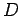Next: 1.4 Organization Up: 1. Introduction Previous: 1.2.2 The Hubbard Model

# 1.3 Methods

Many mathematical models have been introduced to account for the various manifestations of strongly correlated electron systems. These models, while often simple, cannot be fully addressed by traditional perturbative approaches. These standard many-body techniques fail due to the presence of strong electron-electron interactions. While these approaches still provide valuable insight, new models and methods had to be developed to accurately solve the problems.

Diagonalization techniques can provide exact solutions to correlated electron models but they are limited to very small, finite sized systems. This is due to the involved numerical expense which scales exponentially with the number of sites in consideration. Any systems thus investigated will be delimited in size by Moore's law(11). Based on empirical observation, it can be interpreted as a predictive doubling of computational processing power every 18 months, thereby increasing the feasible system size by only a single site every two years. While this is not a universally true prediction it is expected to remain valid for the near future.

One exact diagonalization technique is the Wegner flow equation approach. Here the Hamiltonian is diagonalized by continuous infinitesimal unitary transformations, thus establishing the flow equations. In chapter 2, further details to the approach and its application to the Kondo model are provided.

Possibly the best solution to strongly correlated many-body models is provided by the Quantum Monte Carlo methods. In place of an explicit integration over the configuration space, one introduces a sampling thereof. This results in a significant improvement in computational cost, reducing the scaling to an algebraic one for favorable applications. This improved scalability allows the QMC to treat larger systems, but calculations of dynamical quantities remains problematic. An additional limitation may arise from the so-called 'sign problem'. It manifests itself in a cancellation of QMC contributions due to a minus sign introduced by the co-ordinate exchange of two electrons (Pauli principle). The scaling may thus revert to an exponential one, limiting the QMC beyond the mere computational demands. For a comprehensive overview of the method see (12).

In chapter 3, a QMC calculation is employed to address the one-dimensional Hubbard model. Within a path-integral formalism thedimensional problem is recast as adimensional one, where the fermion interaction has been eliminated at the expense of an additional summation on an auxiliary Ising field. The only approximation within this approach is the assumption of commutation for the interacting and non-interacting parts of the Hamiltonian at short imaginary time intervals. This approximation can be systematically improved asis shortened. A detailed discussion can be found in (13).

The density-matrix renormalization group (DMRG)(14) is another one of the numerical highly exact solvers. It is an application of the standard numerical renormalization group (NRG) to quantum lattice systems. While it does succeed in treating larger system sizes, as well as lower temperatures than the QMC, it remains most applicable in one-dimensional systems. In the DMRG the system's Hilbert space is systematically truncated and the most important states are used to construct most probable wave-functions of the quantum-mechanical system. A full review of the DMRG can be found in (15).

Alongside the numerical techniques, there have also been a wide range of analytical approaches. Amongst these are Bozonization(16) and Function Integral(17) techniques, which will not be further discussed here but are only mentioned for completeness.

In the previous numerical methods, the calculated system sizes were severely restricted, contingent on the computational feasibility. Due to the relatively small system sizes, the solutions exhibit, sometimes severe, finite-size effects. This results in an overestimation of correlations and limits their ability to calculate dynamical and transport properties. Thus arose the need for an approximation which would correctly include local correlations. One such method is the dynamical mean field theory (DMFT) as proposed by Metzner and Vollhardt(18) and Müller-Hartmann(19). The DMFT is a non-perturbative approximation which maps the lattice problem onto that of a single, local impurity, coupled to an effective bath. The resulting impurity problem can once again be solved by exact numerical methods. The problem is effectively divided into two parts: Local-correlations are considered explicitly and the remaining non-local correlations are treated in an effective medium. This mapping was further justified by Metzner and Vollhard when they realized that in the limit of infinite dimensions this approximation becomes exact. It is noteworthy that the nomenclature 'Mean-field' only applies to spacial dependency whereas all temporal fluctuations are preserved. For a complete review of the DMFT see (20) and (21).

The DMFT was widely successful in addressing some of the shortcomings of finite-size calculations. Due to its purely local nature however, it was unable to capture non-local fluctuations - i.e. such as would give rise to spin waves. In an extension, quantum cluster method allow for the explicit inclusion of non-local, spacial fluctuations. In these methods the infinite lattice is now mapped onto a finite size cluster, which in turn is embedded in a dynamical mean-field. Once again, the reader is referred to a full review of these methods by Maier et. al (22) and direct numerical comparison by Biroli and Kotilar(23). This class of solvers has been highly successful in the study of strongly correlated electron-systems, such as highsuperconductors, where the correlations are short ranged.

In chapter 3, a numerically implementable Multi-scale Many-Body approach to strongly correlated electron systems is introduced. It allows for the inclusion of long ranged correlations beyond the reach of traditional cluster methods. This extension to quantum cluster methods approximates correlations on any given length-scale commensurate with the strength of the correlations on the respective scale. Short length-scales are treated explicitly, long ones are addressed at a mean-field level, and intermediate ranged correlations are assumed to be weak and are approximated diagrammatically. To illustrate and test this method, it is applied to the one dimensional Hubbard model. The resulting multi-scale self-energy provides a very good quantitative agreement with substantially more numerically expensive, explicit Quantum Monte-Carlo calculations.Next: 1.4 Organization Up: 1. Introduction Previous: 1.2.2 The Hubbard Model
© Cyrill Slezak